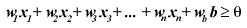2 views

I'm having trouble seeing what the threshold actually does in a single-layer perceptron. The data is usually separated no matter what the value of the threshold is. It seems a lower threshold divides the data more equally; is this what it is used for?

by (108k points)

Perceptrons are the data structures that help to learn for the study of Neural Networking. Think of a perceptron as a node of a vast, interconnected network, sort of like a binary tree, although the network does not necessarily have to have a top and bottom. The links between the nodes of the model, not only show the relationship between the nodes but also transmit data and information, called a signal or impulse. The perceptron is a simple model of a neuron (nerve cell). Since linking the perceptrons into a network is a bit difficult to perform, let's take a perceptron by itself.

A perceptron has a number of external input links, one is the internal input (called a bias), second is a threshold and the last one is the output link. The threshold is the key component of the perceptron. It estimates, based on the inputs, whether the perceptron fires or not. The perceptron takes all of the weighted input values and adds them together. If the sum is above or equal to some value (called the threshold) then the perceptron fires. Otherwise, the perceptron does not. So, it fires whenever the following equation is true (where w represents the weight, and there are n inputs):If you wish to know more about Neural Networking then visit this Neural Network Tutorial.